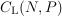# Gaussian feedback MAC

### Linear-feedback sum-capacity for Gaussian multiple access channels

Ehsan Ardestanizadeh, Michele Wigger, Young-Han Kim, and Tara Javidi

The capacity region of the-sender Gaussian multiple access channel with feedback is not known in general. This paper studies the class of linear-feedback codes that includes (nonlinear) nonfeedback codes at one extreme and the linear-feedback codes by Schalkwijk and Kailath, Ozarow, and Kramer at the other extreme. The linear-feedback sum-capacityunder symmetric power constraintsis characterized, the maximum sum-rate achieved by linear-feedback codes when each sender has the equal block power constraint. In particular, it is shown that Kramer's code achieves this linear-feedback sum-capacity. The proof involves the dependence balance condition introduced by Hekstra and Willems and extended by Kramer and Gastpar, and the analysis of the resulting nonconvex optimization problem via a Lagrange dual formulation. Finally, an observation is presented based on the properties of the conditional maximal correlation — an extension of the Hirschfeld-Gebelein-Renyi maximal correlation — which reinforces the conjecture that Kramer's code achieves not only the linear-feedback sum-capacity, but also the sum-capacity itself (the maximum sum-rate achieved by arbitrary feedback codes).